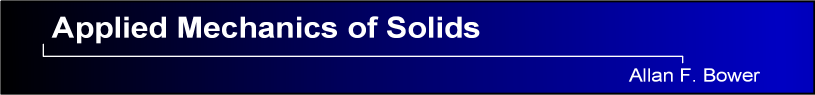Problems for Chapter 10

Approximate Theories for Solids with Special Shapes:

Rods, Beams, Membranes, Plates and Shells

10.6.    Simplified Versions of General Shell Theory10.6.1.    Consider a shell that is so thin that the internal momentsall vanish.  Find the simplified equations of motion for the internal forcesand the transverse forcein terms of relevant geometric parameters.10.6.2.    The figure shows a thin circular plate with thickness h, mass density, Young’s modulus E and Poisson’s ratiothat is simply supported at its edge and is subjected to a pressure distribution acting perpendicular to its surface.  The goal of this problem is to derive the equations governing the transverse deflection of the plate in terms of the cylindrical-polar coordinatesystem shown in the figure.

10.6.2.1.           Write down the position vector of a point on the mid-plane of the undeformed plate in terms of, expressing your answer as components in thebasis.

10.6.2.2.           Calculate the basis vectorsand, expressing your answer as components in the basisshown in the figure.

10.6.2.3.           Find the components of the Christoffel symbolfor the undeformed plate;

10.6.2.4.           Calculate the contravariant components of the metric tensor10.6.2.5.           Find the basis vectorsfor the deformed plate, neglecting terms of order, etc

10.6.2.6.           Show that the curvature tensor has components10.6.2.7.           Express the internal momentsin the plate in terms ofandand its derivatives.

10.6.2.8.           Write down the equations of motion for the plate in terms ofand10.6.2.9.           Hence, show that the transverse displacement must satisfy the following governing equation(c) A.F. Bower, 2008
This site is made freely available for educational purposes.
You may extract parts of the text
for non-commercial purposes provided that the source is cited.
Please respect the authors copyright.